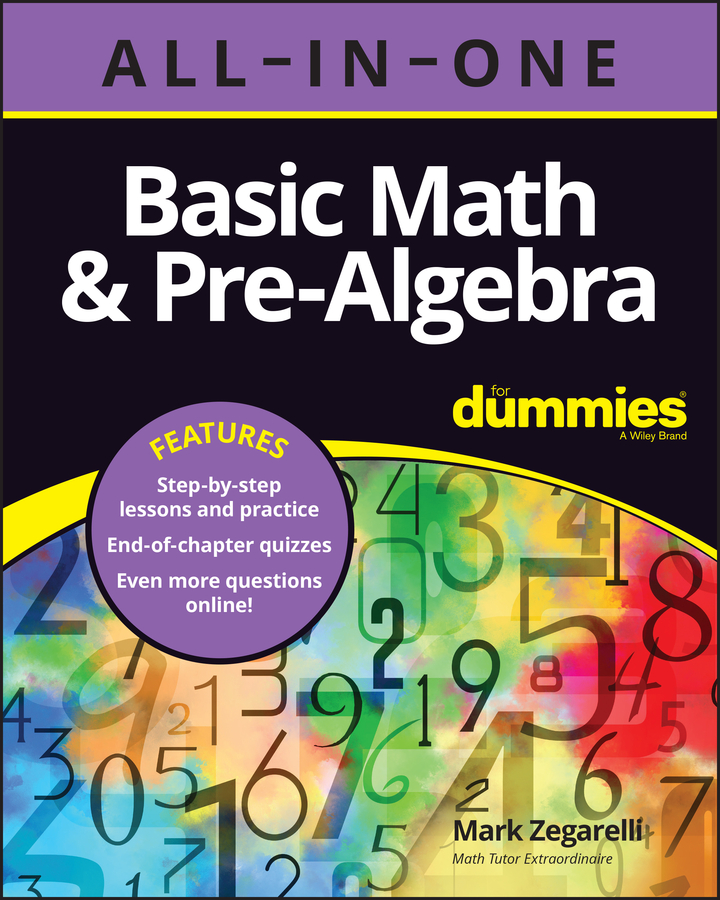##### Basic Math & Pre-Algebra All-in-One For Dummies (+ Chapter Quizzes Online)To solve complex word problems, you use the same skills as when you solve basic word problems, but the calculations become harder. For example, instead of a dress costing an amount such as \$30, which is easy to calculate, it may cost \$29.95. Also, the amount of information in the problem may increase.

A lot of problems that look tough aren’t much more difficult than simple problems. For example, consider this problem:

Aunt Effie has \$732.84 hidden in her pillowcase, and Aunt Jezebel has \$234.19 less than Aunt Effie has. How much money do the two women have altogether?

One question you may have is how these women ever get any sleep with all that change clinking around under their heads. But moving on to the math, even though the numbers are larger, the principle is still the same as in a simpler word problem. Start reading from the beginning: “Aunt Effie has \$732.84. . . .” This text is just information to jot down as a simple word equation:

Effie = \$732.84

Continuing, you read: “. . . Aunt Jezebel has \$234.19 less than Aunt Effie has.” It’s another statement you can write as a word equation:

Jezebel = Effie – \$234.19

Now you can plug in the number \$732.84 where you see Aunt Effie’s name in the equation:

Jezebel = Effie \$732.84 – \$234.19

So far, the big numbers haven’t been any trouble. At this point, though, you probably need to stop to do the subtraction:

\$732.84

– \$234.19

\$498.65

Now you can jot this information down as follows:

Jezebel = \$498.65

The question at the end of the problem asks you to find out how much money the two women have altogether. Here’s how to represent this question as an equation:

Effie + Jezebel = ?

You can plug information into this equation:

Effie \$732.84 + Jezebel \$498.65 = ?

Again, because the numbers are large, you probably have to stop to do the math:

\$732.84

+ \$498.65

\$1,231.49

So, altogether, Aunt Effie and Aunt Jezebel have \$1,231.49.

As you can see, the procedure for solving this problem is basically the same as for a simpler word problem. The only difference is that you have to stop to do some addition and subtraction.